# Combination: Detailed Explanation JEE Notes | EduRev

## JEE : Combination: Detailed Explanation JEE Notes | EduRev

The document Combination: Detailed Explanation JEE Notes | EduRev is a part of the JEE Course Mathematics (Maths) Class 11.
All you need of JEE at this link: JEE

Combination

Each of the different groups or selections which can be made by some or all of a number of given things without reference to the order of the
things in each group is called a combination.

Mathematically The number of combinations of n different things taken r at a time is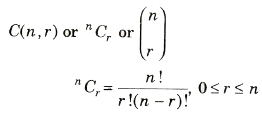Properties of Combination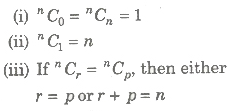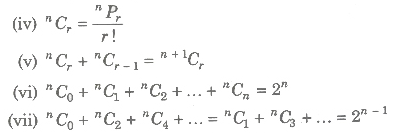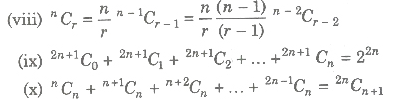Important Results on Combination

• The number of combinations of n different things taken r at a time allowing repetitions is n + r – 1Cr
• The number of ways of dividing n identical things among r persons such that each one gets at least one is n – 1Cr – 1.
• The total number of combinations of n different objects taken r at a time in which
(a) m particular objects are excluded = n – mCr
(b) m particular objects are included = n – mCr – 1
• The total number of ways of dividing n identical items among r persons, each one of whom can receive 0, 1, 2 or more items (≤ n) is n + r – 1Cr – 1
• The number of ways in which n identical items can be divided into r groups so that no group contains less than in items and more than k(m < k) is coefficient of xn in the expansion of (xm + xm + 1 +….+ xk)r.
• The total number of ways of selection of some or all of n things at a time is nC1nC2 +….+ nn1 = 2n — 1.
• The number of selections of r objects out of n identical objects is 1.
• Total number of selections of zero or more objects from n identical objects is n + 1.

Important Points to be Remembered

1. Function

(i) If a set A has m elements and set B has n elements, then

(a) number of functions from A to B is nm
(b) number of one-one function from A to B is nPm, m ≤ n.
(c) number of onto functions from A to B is nm — nC1(n — 1)mnC2(n — 2)m…..; m ≤ n.
(d) number of increasing (decreasing) functions from A to B is nCm, m ≤ n.
(e) number of non-increasing (non-decreasing) functions from A to B is m + n – 1Cm .
(f) number of bijective (one-one onto) functions from A to B is n !, if m = n.

(ii) Number of permutations of n different objects taken r at a time in which m particular objects are always

(a) excluded = n – mPr
(b) included = n – mPr – m x r!

2. Geometry

1. Given, n distinct points in the plane, no three of which are collinear, then the number of line segments formed = nC2.
2. Given. ii distinct paints in the p)ane. in which m are collinear (m ≥ 3), then the number of line segments is (nC2 – mC2) + 1.
3. Given, n distinct points in the plane, no three of which are collinear, then the number of triangle formed = nC3
4. Given, n distinct points in a plane, in which m are collinear (m ≥ 3), then the number of triangle formed = nC3 — mC3
5. The number of diagonals in a n-sided closed polygon = nC2 — n.
6. Given, n points on the circumference of a circle, then
(a) number of straight lines = nC2
(b) number of triangles = nC3
(c) number of quadrilaterals = nC4
7. Number of rectangles of any size in a square of n x n is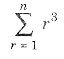and number of square of any size is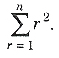.
8. In a rectangle of n x p (n < p), numbers of rectangles of any size is np/4 (n + 1) (p + 1) and number of squares of any size is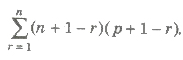9. Suppose n straight lines are drawn in the plane such that no two lines are parallel and no three lines are concurrent, then number of parts which these divides the plane is equal to 1 +∑ n.

3. Prime Factors

Any natural number > 1, can be expressed as product of primes.

• Let n = p1α1 p2α2 p3α3 …. prαr, where
• pi, i = 1, 2, 3, … , r, are prime numbers.
• αi, i = 1, 2, 3, … , r, are positive integers.
1. Number of distinct positive integral divisors of n is (α1 + 1)(α2 + 1)(α3 + 1) … (αr + 1).
2. Sum of distinct positive integral divisors of n is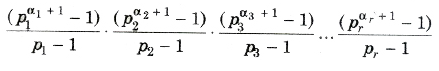3. Total number of divisors of n (excluding 1 and n), is (α1 + 1)(α2 + 1)(α3 + 1) … (αr + 1) – 2.
4. Total number of divisors of n (excluding 1 or n), is (α1 + 1)(α2 + 1)(α3 + 1) … (αr + 1) – 1.
5. The number of ways in which n can be resolved as a product of two factors is
(a) 1/2(α1 + 1)(α2 + 1)(α3 + 1) … (αr + 1) if n is not a perfect square.
(b) 1/2[(α1 + 1)(α2 + 1)(α3 + 1) … (αr + 1) + 1], if n is a perfect square.
6. The number of ways in which n can be resolved into two factors which are prime to each other is 2r – 1, where r is the number of different factors in n.
7. If p is prime and pr divides n!, then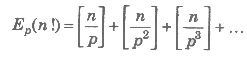4. Integral Solutions

1. The number of integral solutions of x1+ x2 +….+ xr = n, where x1, x2, … xr ≥ 0 is n + r – 1Cr – 1.
2. Number of integral solutions of x1+ x2 +….+ xr = n, where x1, x2, … xr ≥ 1 is n – 1Cr – 1

5. Sum of Digits

1. Sum of the numbers formed by taking all the given n digits = (Sum of all the n digits) x (n — 1)! x (111… 1)n times.
2. The sum of all digits in the unit place of all numbers formed with the help of ai , a2, , an all at a time is (n — 1)!(a1 + a1 + …. + an).
3. The sum of all digits of numbers that can be formed by using the digits a1, a2,… , an(repetition of digits is not allowed (n — 1)! (a1 + a1 + … + an)((10n – 1)/9)

6. Arrangements

1. The number of ways in which m (one type of different things) and n (another type of different things) can be arranged in a row so that all the second type of things come together is n !(m + 1)!.
2. The number of ways in which m (one type of different things) and n (another type of different things) can be arranged in row so that no two things of the same type come together is 2 x m! n!
3. The number of ways in which m (one type of different things) and n (another type of different things) (m ≥ n), can be arranged in a circle so that no two things of second type come together (m – 1)! mPn and when things of second type come together = m! n!
4. The number of ways in which m things of one type and n things of another type (all different) can be arranged in the form of a garland so that all the second type of things come together, is m! n!/2 and if no things of second type come together is, [((m – 1)!mPn)/2]

7. Dearrangements

If n distinct objects are arranged in a row, then the number of ways in which they can be rearranged so that no one of them occupies the place assigned to it is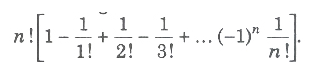8. Selection

1. The total number of ways in which it is possible to make a selection by taking some or all the given n different objects is
nC1nC2 + …. + nCn = 2n – 1
2. If there are m items of one kind, n items of another kind and so on. Then, the number of ways of choosing r items out of these items = coefficient of xr in
(1 + x + x2 + …. + xm)(1 + x + x2 + …. + xn)
3. If there are m items of one kind, n items of another kind and so on. Then, the number of ways of choosing r items out of these items such that at least one item of each kind is included in every selection = coefficient of xr in
(x + x2 + …. + xm)(x + x2 + …. + xn)….
4. The number of ways of selecting r items from a group of n items in which p are identical, is
n – PCrn – PCr – 1n – PCr – 2 + … + n – PC0, if r ≤ P and n – PCrn – PCr – 1n – PCr – 2 + … + n – PCr – p, if r > P
5. The number of ways in which n identical things can be distributed into r different groups is n + r – lCr – 1, or n – 1Cr – 1 according as blanks groups are or are not admissible.
6. The number of ways of answering one or more of n questions is 2n – 1.
7. The number of ways of answering one or more n questions when each question has an alternative = 2n
8. n! + 1 is not divisible by any natural number between 2 and n.
9. If there are 1 objects of one kind, m objects of second kind, n objects of third kind and so on. Then, the number of possible arrangements of r objects out of these objects = Coefficient of xr in the expansion of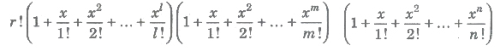Offer running on EduRev: Apply code STAYHOME200 to get INR 200 off on our premium plan EduRev Infinity!

## Mathematics (Maths) Class 11

158 videos|179 docs|161 tests

,

,

,

,

,

,

,

,

,

,

,

,

,

,

,

,

,

,

,

,

,

;